# Kolmogorov-Smirnov Test (not on midterm!)

## Kolmogorov-Smirnov Test

Population: $$Y \sim$$ some population distribution with c.d.f $$F$$

Sample: n i.i.d from population, $$Y_1, \ldots, Y_n$$

Parameter: Whole CDF

Null hypothesis: $$H_0: F = F_0$$, versus $$H_A: F \ne F_0$$

## Kolmogorov-Smirnov Test

Test statistic

$D(F_0) = \sup_{y} \left| \hat{F}(y) - F_0(y) \right|$

where $$\hat{F}(y)$$ is the empirical cumulative distribution function:

$\hat{F}(y) = \frac{1}{n}\sum_{i= 1}^{n} \pmb{1}\left\{ Y_i \le y \right\}$

and $$F_0$$ is the cumulative distribution function for the null hypothesized distribution.

## ECDF: Example

Sample values: 1.8, 2.2, 2.7, 5.7, 6.9, 7.4, 8.1, 8.7, 9 and 9.5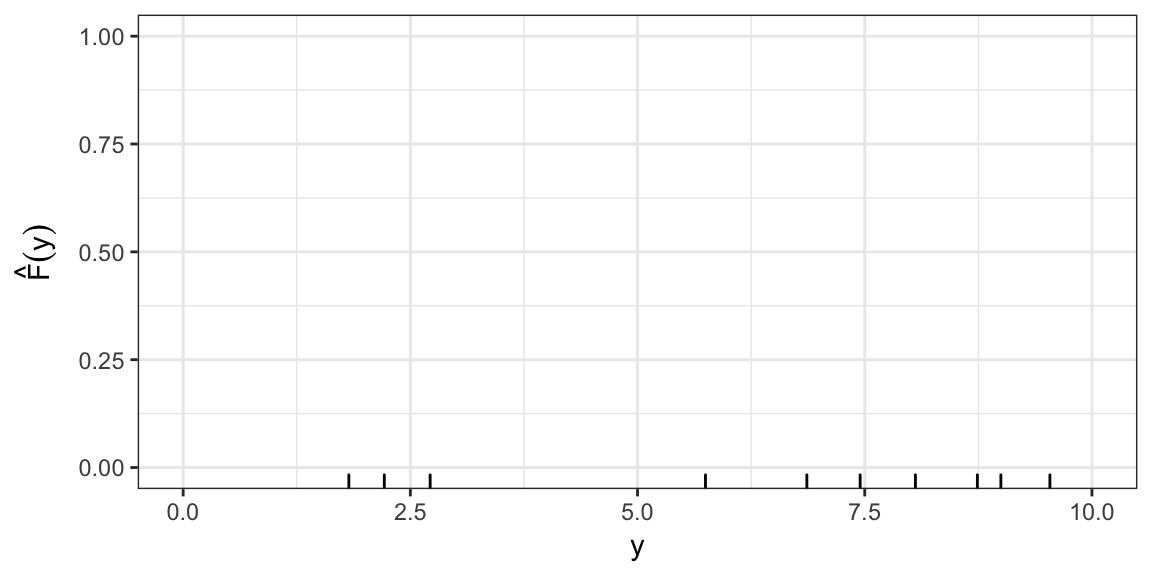## KS test statistic: Uniform(0, 10)

Say, $H_0: F(Y) = \begin{cases} 0, & y \le 0 \\ \frac{y}{10}, & 0 < y \le 10 \\ 1, & y > 10 \end{cases}$

I.e. $$H_0: Y \sim \text{Uniform}(0, 10)$$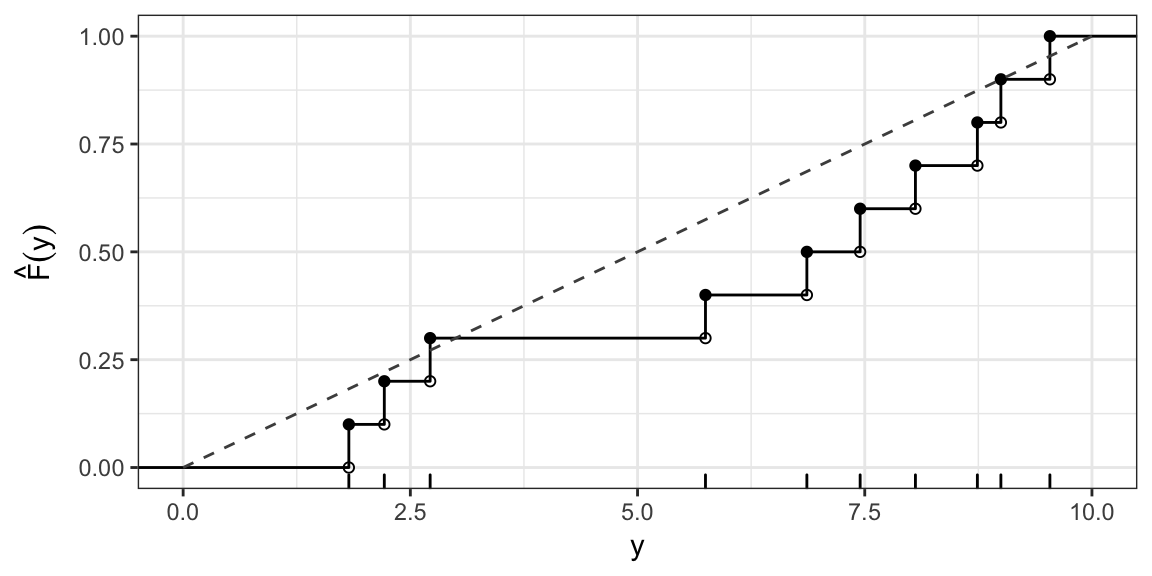## KS test statistic: Uniform(0, 10) cont.

$$D(F_0) = \sup_{y} \left| \hat{F}(y) - F_0(y) \right| \approx 0.29$$ (occurs at $$y$$ just less than 6.9)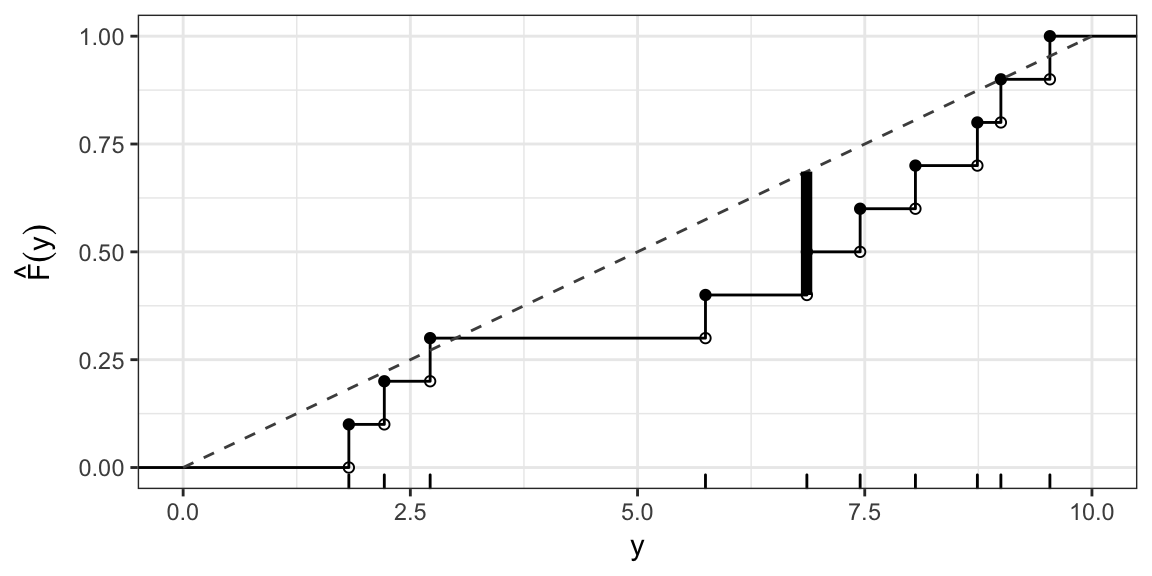## KS test statistic: Normal(5, 6.25)

$$H_0: Y \sim \text{Normal}(5, 6.25)$$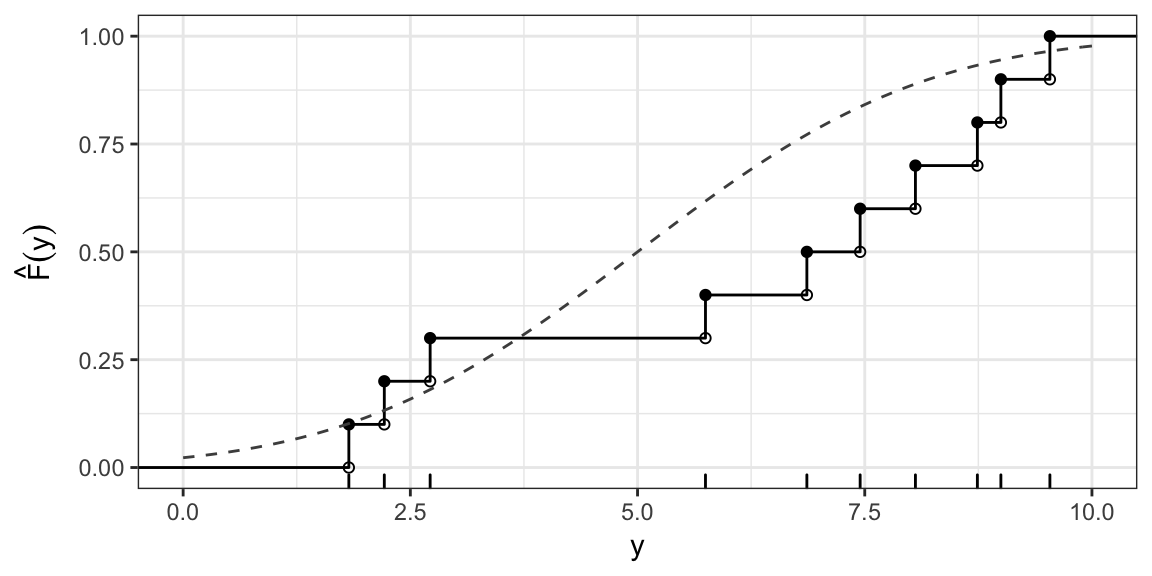## KS test statistic: Example cont.

$$D(F_0) = \sup_{y} \left| \hat{F}(y) - F_0(y) \right| \approx 0.37$$ (occurs at $$y$$ just less than 6.9)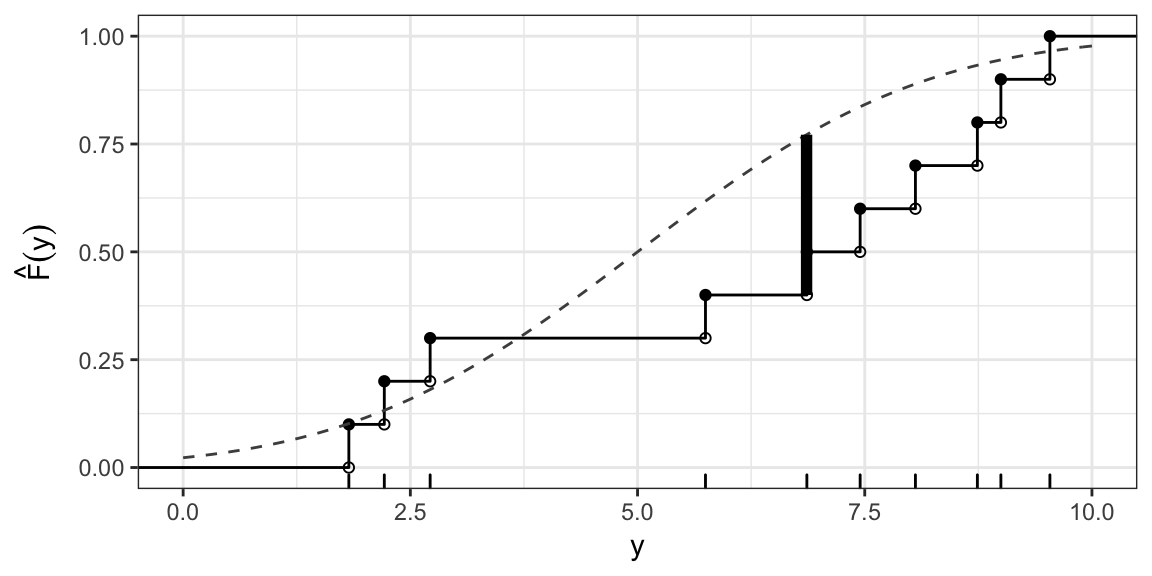## Reference Distribution?

$\sqrt{n} D(F_0) \rightarrow_d K$ where $$K$$ is the Kolmogorov Distribution.

Reject $$H_0$$ for large values of $$\sqrt{n} D(F_0)$$.

## In R

ks.test(x = y, y = punif, min = 0, max = 10)
##
##  One-sample Kolmogorov-Smirnov test
##
## data:  y
## D = 0.28632, p-value = 0.3209
## alternative hypothesis: two-sided

## One sided tests

Lesser alternative: $H_A: F < F_0, \text{ i.e. } F(y) < F_0(y) \text{ for all } y$

Test statistic $D^-(H_0) = \sup_y (F_0(y) - \hat{F}(y))$

Greater alternative: $H_A: F > F_0, \text{ i.e. } F(y) > F_0(y) \text{ for all } y$

Test statistic $D^+(H_0) = \sup_y (\hat{F}(y) - F_0(y) )$

## One sided tests are hard to interpret

Example based on simulated data. $$H_0: Y \sim N(0, 100)$$

n <- 20
y <- rnorm(n, 0, 1)

For greater alternative: $$H_A: F_Y(y) > \Phi(y; 0, 100)$$ where $$\Phi(y; \mu, \sigma^2)$$ is the c.d.f of the Normal$$(\mu, \sigma)$$.

ks.test(y, pnorm, 0, 10, alternative = "greater")
##
##  One-sample Kolmogorov-Smirnov test
##
## data:  y
## D^+ = 0.42016, p-value = 0.000513
## alternative hypothesis: the CDF of x lies above the null hypothesis

## One sided tests are hard to interpret

For lower alternative: $$H_A: F_Y(y) < \Phi(y; 0, 100)$$:

ks.test(y, pnorm, 0, 10, alternative = "less")
##
##  One-sample Kolmogorov-Smirnov test
##
## data:  y
## D^- = 0.44858, p-value = 0.0001717
## alternative hypothesis: the CDF of x lies below the null hypothesis

## One sided tests

The combination of the two one-sided alternatives, does not cover all the possibilities for which the null hypothesis is false.

This makes it very hard to interpret one-sided KS tests - i.e. don’t do a one-sided test.

## Estimating parameters

The KS test should only be used if you can completely specify $$F_0$$, the population distribution under the null hypothesis.

You should not estimate parameters from the data then do the test.

Kind of like trying to test $$H_0: \mu = \overline{Y}$$, you’ll rarely reject.

## Next time…

After midterm: what if distribution is discrete?